### 东风悦达起亚 赛拉图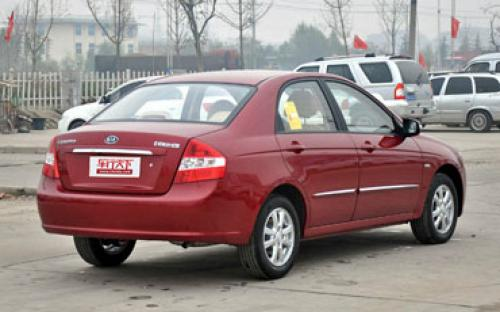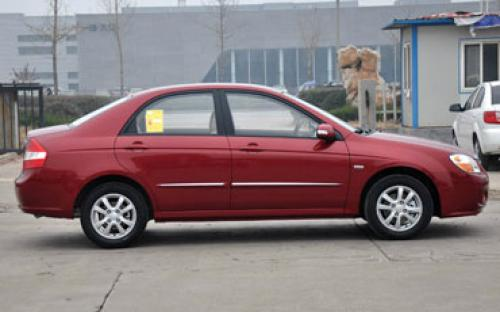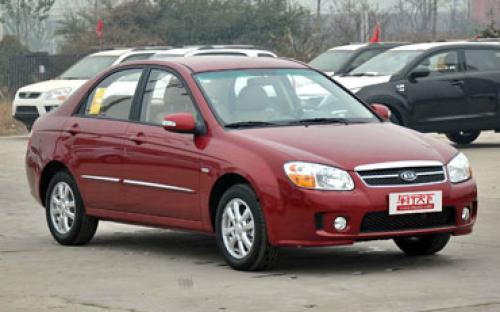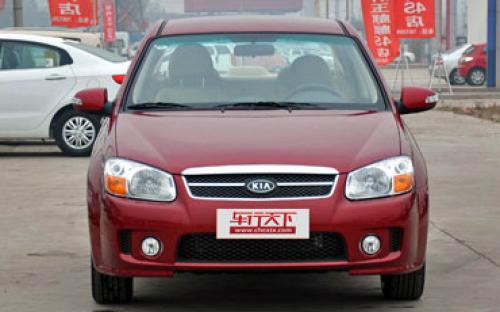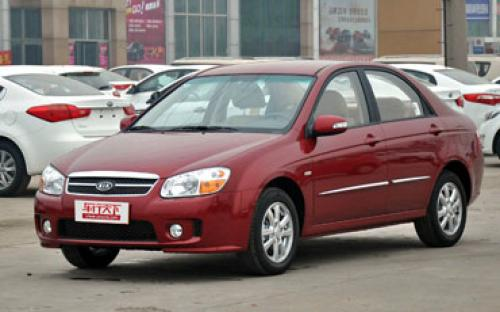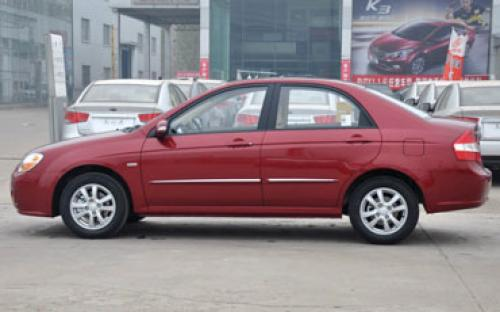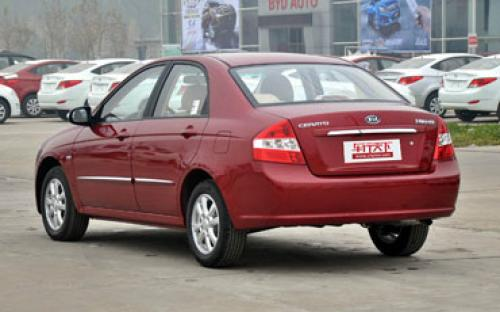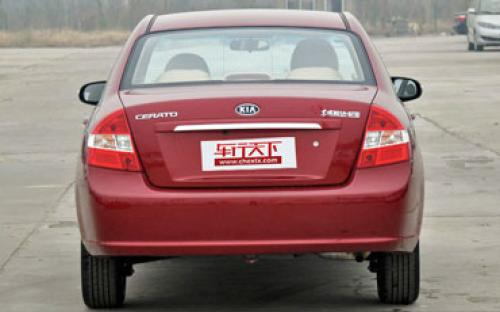12 种颜色可选2012款最低售价：8.88 万元起

4500(mm)1735(mm)1470(mm)##### 配置亮点：
• 胎压监测装置

• ISOFIX儿童座椅接口

• 车身稳定控制(ESC/ESP/DSC等)

• 电动天窗

• 定速巡航

• 后倒车雷达

• 真皮座椅

• GPS导航系统

• 氙气大灯

• 后视镜加热

• 提交

东风悦达起亚 赛拉图 绕车实拍• 东风悦达起亚 赛拉图 在售车型

排量 车型 厂商指导价 本地最低报价 购车工具
1.6L
1.6L MT GL 5挡手动
8.98万
7.23万
1.75万

1.6L AT GL 4挡自动
10.08万
10.08万

1.6L MT GLS 5挡手动
10.38万
10.38万

1.6L AT GLS 4挡自动
11.48万
11.48万

1.8L MT TOP 5挡手动
12.38万
10.63万
1.75万

1.8L AT TOP 4挡自动
13.38万
11.63万
1.75万

1.8L
1.6L MT GL 5挡手动
8.98万
7.23万
1.75万

1.6L AT GL 4挡自动
10.08万
10.08万

1.6L MT GLS 5挡手动
10.38万
10.38万

1.6L AT GLS 4挡自动
11.48万
11.48万

1.8L MT TOP 5挡手动
12.38万
10.63万
1.75万

1.8L AT TOP 4挡自动
13.38万
11.63万
1.75万

东风悦达起亚 赛拉图 经销商

查看更多 >>

### 东风悦达起亚 赛拉图 动力加速

赛拉图 0-100公里加速时间分布在 0.0-秒 属于 超跑级

动力级别 加速时间 车型

东风悦达起亚 赛拉图 视频

东风悦达起亚 赛拉图 新闻资讯

# 走运动路线 天下第一城试驾赛拉图1.6L

车系文章 超过4314次关注

前两天在东风悦达起亚的4S店为大家介绍了静态的赛拉图，今天汽车之家编辑应东风悦达起亚的邀请参加了赛拉图的测试联谊会。下面编辑就为大家报一报流水帐，让大家动...

# 10月岛城紧凑型车上牌量前十名—赛拉图

车系文章 超过2538次关注

赛拉图作为起亚的明星车型之一，其延续了起亚的低耗能，高环保特点，自上市以来，该款车一直受到车迷们的好评。新赛拉图在保留原有CVVT发动机动力优势的同时，新赛...

# 风尚升级 东风悦达起亚赛拉图暮色版上市

车系文章 超过2901次关注

近日，东风悦达起亚旗下中级车赛拉图增推暮色版，即日起在全国范围内正式接受订购。此次赛拉图的改款，在原有米色内饰的基础上，追加暮色版内饰，是继07年赛拉图改...

# 汽车投诉榜公布 合资品牌是投诉主力

车系文章 超过2052次关注

本期(7月1日-7月7日)中国汽车投诉排行榜显示，投诉量前十名的车企变化不大，只是自主品牌比亚迪进入第十名。紧凑型车投诉排行榜中，福克斯以153宗投诉高居榜首，V3...

# 合资车型导购 起亚赛拉图

车系文章 超过2949次关注

东风悦达起亚赛拉图长宽高：4350/1735/1470（mm）轴距：2610mm优点：空间宽敞维修便宜缺点：变速器表现不足

# 2012款赛拉图购车手册 推荐1.6L GL MT

车系文章 超过2449次关注

2012款赛拉图在去年广州车展后悄悄上市，共推出两款排量的6款车型，其中包括1.6L排量的4款车型和1.8L排量的2款车型，新款车型较老款车型有6项升级，售价为8.98万-11.38万元。

猜你喜欢

﻿
• 快速找车
• 选择品牌
• 选择品牌
• A  奥迪
• A  阿斯顿·马丁
• A  阿尔法·罗密欧
• B  宝沃
• B  布加迪
• B  巴博斯
• B  保时捷
• B  宾利
• B  奔驰
• B  宝马
• B  本田
• B  别克
• B  标致
• B  比亚迪
• B  宝骏
• B  北汽制造
• B  北汽新能源
• B  北汽幻速
• B  北汽威旺
• B  北京汽车
• B  奔腾
• B  北汽绅宝
• C  长安
• C  长安商用
• C  长城
• C  昌河
• D  大众
• D  道奇
• D  DS
• D  东南
• D  东风风神
• D  东风风行
• D  东风小康
• D  东风风度
• D  东风
• F  福特
• F  丰田
• F  菲亚特
• F  法拉利
• F  福田
• F  福迪
• F  福汽启腾
• G  观致
• G  广汽传祺
• G  广汽吉奥
• G  GMC
• H  红旗
• H  汉腾汽车
• H  哈弗
• H  哈飞
• H  海格
• H  海马
• H  华颂
• H  黄海
• H  华泰
• H  恒天
• J  吉利汽车
• J  捷豹
• J  Jeep
• J  江淮
• J  江铃
• J  金杯
• J  九龙
• J  金旅
• K  凯翼
• K  凯迪拉克
• K  克莱斯勒
• K  科尼塞克
• K  卡威
• K  开瑞
• L  路虎
• L  林肯
• L  劳斯莱斯
• L  兰博基尼
• L  雷克萨斯
• L  铃木
• L  雷诺
• L  理念
• L  力帆
• L  莲花汽车
• L  猎豹
• L  路特斯
• L  陆风
• M  马自达
• M  MG
• M  MINI
• M  玛莎拉蒂
• M  摩根
• M  迈凯轮
• N  纳智捷
• O  欧宝
• O  讴歌
• O  欧朗
• Q  奇瑞
• Q  起亚
• Q  启辰
• R  日产
• R  荣威
• R  瑞麒
• S  三菱
• S  斯威汽车
• S  萨博
• S  smart
• S  斯柯达
• S  斯巴鲁
• S  思铭
• S  双龙
• S  上汽大通
• S  双环
• T  特斯拉
• T  腾势
• W  沃尔沃
• W  五菱汽车
• W  五十铃
• W  威兹曼
• W  威麟
• X  现代
• X  雪佛兰
• X  雪铁龙
• X  西雅特
• Y  一汽
• Y  英菲尼迪
• Y  英致
• Y  依维柯
• Y  野马汽车
• Y  永源
• Z  众泰
• Z  中华
• Z  中兴
• Z  知豆
• 选择车系
• 选择车系
• 车型对比
• 选择品牌
• 选择品牌
• A  奥迪
• A  阿斯顿·马丁
• A  阿尔法·罗密欧
• B  宝沃
• B  布加迪
• B  巴博斯
• B  保时捷
• B  宾利
• B  奔驰
• B  宝马
• B  本田
• B  别克
• B  标致
• B  比亚迪
• B  宝骏
• B  北汽制造
• B  北汽新能源
• B  北汽幻速
• B  北汽威旺
• B  北京汽车
• B  奔腾
• B  北汽绅宝
• C  长安
• C  长安商用
• C  长城
• C  昌河
• D  大众
• D  道奇
• D  DS
• D  东南
• D  东风风神
• D  东风风行
• D  东风小康
• D  东风风度
• D  东风
• F  福特
• F  丰田
• F  菲亚特
• F  法拉利
• F  福田
• F  福迪
• F  福汽启腾
• G  观致
• G  广汽传祺
• G  广汽吉奥
• G  GMC
• H  红旗
• H  汉腾汽车
• H  哈弗
• H  哈飞
• H  海格
• H  海马
• H  华颂
• H  黄海
• H  华泰
• H  恒天
• J  吉利汽车
• J  捷豹
• J  Jeep
• J  江淮
• J  江铃
• J  金杯
• J  九龙
• J  金旅
• K  凯翼
• K  凯迪拉克
• K  克莱斯勒
• K  科尼塞克
• K  卡威
• K  开瑞
• L  路虎
• L  林肯
• L  劳斯莱斯
• L  兰博基尼
• L  雷克萨斯
• L  铃木
• L  雷诺
• L  理念
• L  力帆
• L  莲花汽车
• L  猎豹
• L  路特斯
• L  陆风
• M  马自达
• M  MG
• M  MINI
• M  玛莎拉蒂
• M  摩根
• M  迈凯轮
• N  纳智捷
• O  欧宝
• O  讴歌
• O  欧朗
• Q  奇瑞
• Q  起亚
• Q  启辰
• R  日产
• R  荣威
• R  瑞麒
• S  三菱
• S  斯威汽车
• S  萨博
• S  smart
• S  斯柯达
• S  斯巴鲁
• S  思铭
• S  双龙
• S  上汽大通
• S  双环
• T  特斯拉
• T  腾势
• W  沃尔沃
• W  五菱汽车
• W  五十铃
• W  威兹曼
• W  威麟
• X  现代
• X  雪佛兰
• X  雪铁龙
• X  西雅特
• Y  一汽
• Y  英菲尼迪
• Y  英致
• Y  依维柯
• Y  野马汽车
• Y  永源
• Z  众泰
• Z  中华
• Z  中兴
• Z  知豆
• 选择车系
• 选择车系
• 选择车型
• 选择车型
• 意见反馈